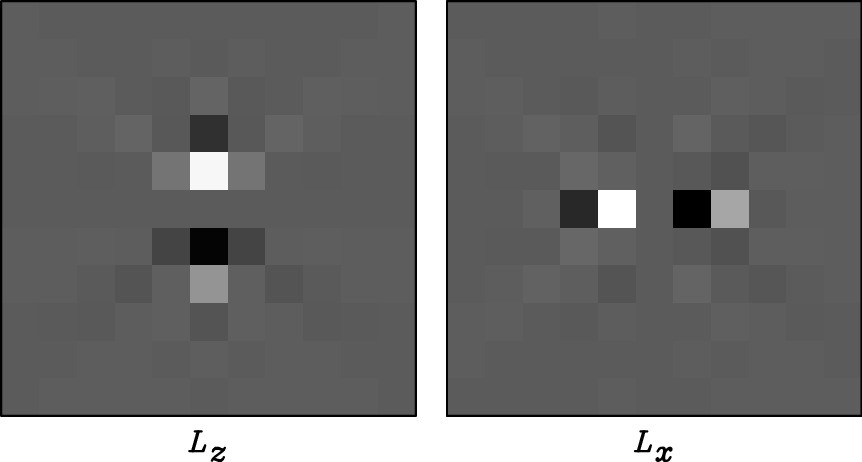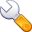Elastic wave-mode separation for VTI mediaNext: Operator truncation Up: Operator properties Previous: Operator orders

## Operator size and compactness

Figure 3 shows the derivative operators of,,, andorders in theanddirections for isotropic and VTI (=0.25,=-0.29) media. As we can see, isotropic operators become longer when the order of accuracy is higher. Anisotropic operators, however, do not change much in size. One can see that the central parts of the anisotropic operators look similar to their corresponding isotropic operators and change with the order of accuracy; while the outer parts of these anisotropic operators all look similar, and do not change much with the order of accuracy. This indicates that the central parts of the operators are determined by the order of accuracy, while the outer parts are representation of the degree of anisotropy.

Figure 4 shows anisotropic derivative operators with same order of accuracy (order in space) for three VTI media with different combinations ofand. These operators have similar central parts, but different outer parts. This result is consistent with the previous observation that the central part of an operator is determined by the order of accuracy, and the outer part is controlled by anisotropy parameters.

Figure 5(a) shows the influence of approximation to finite difference (andorder, Figures 3(h) and fig:oporder-mop2). The anisotropic'' part (diagonal tails'') are almost the same, and the difference comes from the central part. Figure 5(b) shows the difference between operators with different anisotropy (Figures 4(a) and fig:aniopsize-mop1-order8). The difference mainly lies in the tails'' of the operators.

A comparison between Figures 4(a) and fig:aniopsize-mop1-order8 shows that when one has large difference betweenand, the operator is big in size and when the difference ofandstays the same, the parameteraffects the operator size. A comparison between Figures 4(b) and fig:aniopsize-mop2-order8 shows that when the difference betweenandbecomes smaller anddoes not change, the operator get smaller in size. This result is consistent with the polarization equation for VTI media with weak anisotropy (Tsvankin, 2005):(10)

whereandare vertical P and S wave velocities,is the phase angle, andis the P wave polarization angle. This equation demonstrates the deviation of anisotropic polarization vectors with the isotropic ones: difference ofand(which is approximatelyfor weak anisotropy) and the parametercontrol the deviation offromand therefore the size of the anisotropic derivative operators.mop0-order8,mop1-order8,mop2-order8
Figure 4.order anisotropic pseudo derivative operators for three VTI media: a)=0.25,=-0.29, b)=0.54,=0, and c)=0.2,=0.diff0,diff1
Figure 5.
(a) The difference between theandorder operators (Figures 3(h) and fig:oporder-mop2) for a VTI medium with anisotropy=0.25,=-0.29 in theanddirections. (b) The difference between theorder anisotropic operators for a VTI medium with anisotropy=0.25,=-0.29 (Figure 4(a)) and a VTI medium with anisotropy=0.54,=0 (Figure 4(b)).Elastic wave-mode separation for VTI mediaNext: Operator truncation Up: Operator properties Previous: Operator orders

2013-08-29